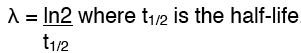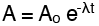The nuclei of radioactive atoms are unstable. That may be because they are too bog, have too many protons or too many neutrons. To become more stable they might emit an alpha particle, beta plus or beta minus or others. There is more detail about this here.

We have some understanding of why some atoms are unstable and break down but we cannot predict when, or even if, a particular atom will break down and emit a particle or energy. If we have a large number of atoms we can predict roughly how many will break down in a given time but not which ones. The process is predictable with large numbers but quite random with individual atoms.

It is just like spinning a coin. If we do this 100 times we are fairly sure we will get about 50 heads but it is unlikely to be exactly 50. If we spin a coin once there is no way to accurately predict the outcome.

Some Maths

The mathematical expression of the activity of a source of radioactivity is

dN/dt  which represents the number of atoms of a particular radioactive isotope emitting a radioactive particle at a particular instant in time. But the more atoms we have the more chance there is of more breaking down so :

dN/dt is proportional to N the number of atoms we have. We make this an equal sign by introducing a constant so

dN/dt = - λN where λ is called the decay constant. The minus sign is there because in decay the number of atoms is falling.

N is continuously falling as atoms decay to form different elements. Therefore it follows that the number decaying every second dN/dt will also be falling. This connection where the rate of change of a quantity depends on the quantity itself is called "exponential"

Graphs of radioactive decay and measuring half life

If we plot a graph of how the amount of radioactivity changes with time we get something like this:

Because of the random nature of decay, the curve of the graph plotting the the number of atoms remaining against time, is not always smooth.  Once the points are plotted a smooth line is drawn to even out the irregularities.

The half life is the time taken for the amount of radioactivity to fall to half of its original value. There is no such thing as a full life. The amount of radioactivity fall and falls again but never really completely disappears (although the level may drop so far that it is insignificant).

The half life should be measured in several places on the graph and an average taken. This again is because radioactive decay is random and subject to slight unpredictable variation.

Examples finding and using half life

Because of the random nature of radioactive decay one value of the half-life is not reliable, so we take an average of several.

Those we have taken here are: 18.4! 21.6! 19.5! 21.8 which to 3 significant figures is 20.3 seconds.

From the half-life we can calculate the decay constant:Working out how radioactive something will be in the future

Knowing a current value of activity we can easily calculate itsʼ value several half lives later by halving that value several times: For example if the activity now is 800 counts per second and the half-life is one year then:

• Now 800
• 1yr 400
• 2yr 200
• 3yr 100
• 4yr 50

and so on, similarly, if the specimen we have is several years old we can work out that the activity:

• one year ago was 1600
• two years ago 3200,
• three years ago 6400 and so on.

The maths is a bit more difficult if we want to calculate the activity over a fractional number of half lives, for example in 6 months or 2.3 years ago, but we can do it. Let's use the same example. If the activity is 800 counts per second and the half life 1 year, then after si months we know the activity must have fallen but not so far as 400 because that is the likely activity after 1 year. The answer is not 600 because it is not a linear relationship but it might not be too far from that.

To do this we use the equation:where A is the activity at time t,  Ao is the activity at the start,  λ is the decay constant. Remember that:so λ = ln2/1 = 0.693 per year.

so A = 800 x  2.718 to the power of -0.693 x 0.5    (0.5 because 6 months is half of a year)

= 800 x 0.717 = 574  (to 3 significant figures)

There are more useful pages about different aspects and uses of radioactivity here: